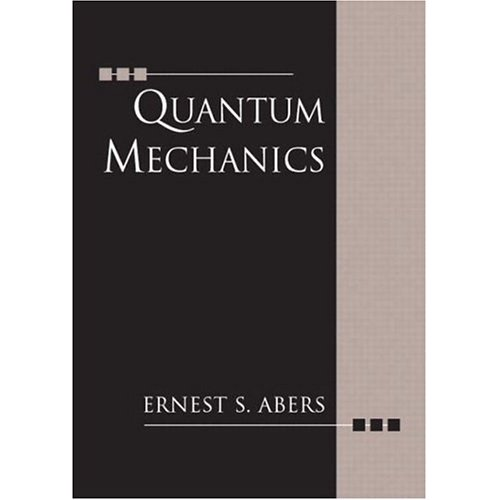Misak Sargsian

M,W 5:00-6:15pm, CP103

Office Hours M,W - 3:30-4:30pm, CP224/zoom, sargsian@fiu.edu, 305-348-3954

`Lecture 1- Classical Physics: (1)Mechanics and Newton's Laws  (2) Principles vs Laws (3) Basic Quantities of the nature (4) Lagrangian, Action, Least Action Principle (5)Hamiltonian Lecture 2 - Classical Physics: (1) Symmetries, (2)Transformations & Operators (3) Elements of group theory, (4) Generators and Algebra(5)Example of Space Translation and RotationLecture 3 - Foundation of Quantum MechanicsPlanck Paper (1)Complex Vector States in the Hilbert Space (2)Linear Operators (3)Relating above to physical observables (4)Correspondence Principle Lecture 4 - Schroedinger Equation 1)Time-Translation of the state vectors and Hamiltonian 2) Shcroedinger Equation and canonical commutators 3)Planck constant and natural units 4)Uncertainty principle 5) Space translation of state vectors and momentum operator 6) Wave functions and Shroedinger wave equation 7) Continuity equation - no probability is lost in QMLecture 5 - Stationary States 1)Seven Pillars of Wisdom of Schroedinger Equation 2) Virial TheoremLecture 6 - One Dimensional Examples: (1) Using symmetry properties of Hamiltonian to     solve Schroedinger equations(2) Two state systems(3) Infinite square well (4) Finite size one-dimensional square well(5) Delta function type potentials(6) Canonical Quantization(7) Harmonic Oscillators`
` Lecture 7- Three Dimensions with Spherical  Symmetry (1)Orbital Angular momentum; (2) What it means to be spherically symmetric in QM  (3) Properties of Angular momentum operator (4) Schroedinger Equation for Spherical Symmetry case  (5) Runge-Lenz vector in quantum mechanics  (6) Hydrogenlike Atoms  (7) Radial Wave functions of Hydrogen Atom`
` Lecture 8- Symmetry and Spin                            (1)Spin and Rotations  (2)Generators of  rotation in the spinor space (3) Spin wave functions (4) Total angular momentum  (5)Addition of Angular Momenta (6) Clebsch-Gordan Coefficients (7) Example of Deuteron Wave function`
```Lecture 9 - Approximation Methods of Bound State
(I)Bound  State Perturbation Theory (1)The Perturbation Expansion (2)Example:Harmonic Oscillator (3)Fine Structure of Hydrogen Atom     (3.1) The Spin-Orbit Coupling Correction     (3.2) The Relativistic Kinetic Energy Correction  (4) The Hyperfine Structure of the Hydrogen Atom (5) Other Atoms(6) Atomic Clocks (II) The Variational Method(1) The General method(2) Application to the Helium Atom (III)Molecules(1)The Born-Oppenheimer Approximation(2)Application to Hydrogen Molecular Ion   (IV) WKB Approximation     Lecture 10- Potential Scattering             (1)Kinematics, Setting up the scattering problem  (2)The Scattering Amplitude(3) Born Approximation, Yukawa and Coulomb interactions(4)The Optical Theorem(5) Partial Waves     (5.1) Expansion of a Plane Wave in a Legandre Series     (5.2) Partial wave expansion of the scattering amplitude     (5.3) Calculation of the Phase Shift(6) The Radial Wave function    (6.1) The integral Equation    (6.2) Partial Wave Green's Functions    (6.3) Scattering by an Impenetrable Sphere Lecture 11- Quantum Mechanics of Many-Body Systems(1)Nonrelativistic Identical-Particle Systems(2) Creation and Annihilation of Bosons(3) Creation and Annihilation of Fermions (4) Bosonic Gas(5) Fermionic Gas(6) From White Dwarfs to Neutron Stars ```
 ? Information.Primary Textbook: "Quantum Mechanics" Ernest S. Abers
"Quantum Mechanics" L.Landau & E.Lifshitz
"Lectures on Quantum Mechanics" Steven Weinberg

Two  pieces of the Final Grade:

(evaluated by the fraction of problems solved) 25%
Take Home Midterm Exam  (30%) (Sept 29)
Final Cumulative In Class Exam (45%) 12/6/2021
(5-7pm, CP103)

Homework Assignments:

HW1.pdf ( due  Sep 8 )

HW2.pdf (due  Sep 15 )

HW3.pdf (due  Sep 29 )

Exam1.pdf (due Oct. 4)

HW4.pdf (due   Oct 11  )

HW5.pdf (due Oct 20  )

HW6.pdf (due  Nov 1   )

HW7.pdf (due  Nov 15  )

HW8.pdf (due   Nov 22 )

HW9.pdf (due   Nov 30 )

HW10.pdf (due  Dec 10 )

COVID Guidlines:

Some policies:  No unjistifed absences, and more than 50% attendances
are required for receiving a final grade.